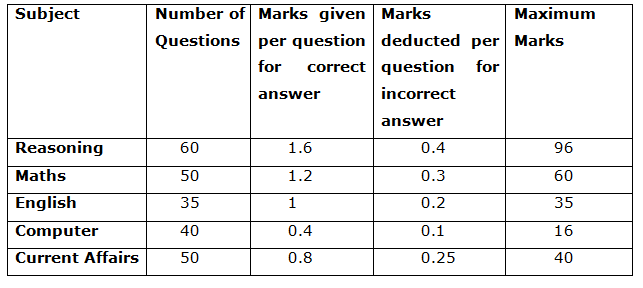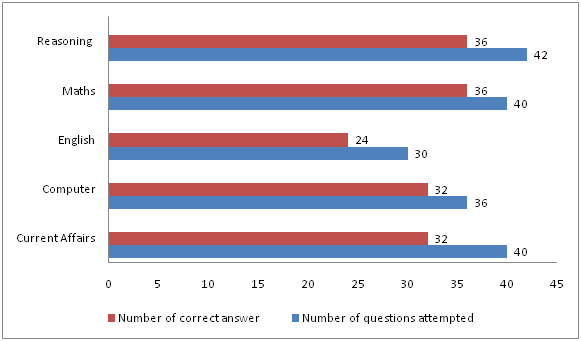# SBI Clerk Mains Quantitative Aptitude (Day-02)

Dear Aspirants, Our IBPS Guide team is providing new series of Quantitative Aptitude Questions for SBI Clerk Mains 2020 so the aspirants can practice it on a daily basis. These questions are framed by our skilled experts after understanding your needs thoroughly. Aspirants can practice these new series questions daily to familiarize with the exact exam pattern and make your preparation effective.

Start Quiz

Ensure Your Ability Before the Exam – Take SBI Clerk 2020 Mains Free Mock Test

Data Interpretation

Directions (1 – 5): Study the following information carefully and answer the questions given below.

The bar graph shows the number of questions attempted by a student in different subjects in an exam conducted by IBPS and the table given below shows the name of subjects, its maximum marks, marks given for each correct answer, and mark deducted for each incorrect answer.Note:

: Percentage of marks obtained in a particular subject = (marks obtained/maximum marks) * 100

: No mark is deducted for the un-attempted question.

1) If minimum 60% of the marks are required to pass in each subject then find the number of subjects in which the student has failed.

a) 0

b) 1

c) 2

d) 3

e) 4

2) The total number of incorrect answers given by the students is approximately what percent of the total number of questions attempted by the student?

a) 12%

b) 15%

c) 18%

d) 20%

e) 22%

3)

Quantity 1: Average of marks obtained in English and Computer.

Quantity 2: One-fourth of sum of marks obtained in Maths and Current Affairs.

a) Quantity I > Quantity II

b) Quantity I ≥ Quantity II

c) Quantity I = Quantity II

d) Quantity I < Quantity II

e) Quantity I ≤ Quantity II

4) Find the percentage of marks obtained by the student in reasoning.

a) 47.5%

b) 62.5%

c) 52.5%

d) 57.5%

e) 67.5%

5) In which subject the ratio of marks deducted for incorrect answer to the marks obtained for correct answer is least?

a) Reasoning

b) Maths

c) English

d) Computer

e) Current Affairs

Applications Sums

6) A part of Rs. 38,800 is lent out at 6% per six months. The rest of the amount is lent out at 5% per annum after one year. The ratio of interest after 3 years from the time when first amount was lent out is 5: 4. Find the second part that was lent out at 5%.

a) Rs. 28800

b) Rs. 28400

c) Rs. 29800

d) Rs. 28400

e) None of these

7) A bank decides to lend Rs. 10 crore through three types of loans namely Personal loan, Home loan and Car loan. The interest rates per annum as well as the bad debt as a percentage of the money lent in each type of mentioned loan is shown in the table given below. Bad debt is a debt that does not give any return and cannot be recovered.

Personal            15%                      5%

Home                 10%                      3%

Car                      9%                       2%

In which of the following ways should the Bank lend Rs. 10 crore such that it maximizes it’s return on the money lent for a period of 1 year?

a) Bank should lend Rs. 5 crore as home loan, Rs. 3 crore as personal loan and the remaining money as car loan.

b) Bank should lend Rs. 5 crore as personal loan and Rs. 5 crore as car loan.

c) Bank should lend an equal amount through all the three types of loans.

d) Bank should lend Rs. 10 crore as car loan.

e) Bank should lend Rs. 10 crore as personal loan.

8) A, B and C started a business by investing Rs. x, Rs. x + 8000 and Rs. x + 18000 respectively. After 6 months, A withdraws Rs. 2000. And after another 2 months, B invested Rs. 5000 more. Find the value of x, if the share of A, B and C at the end of the year is in the ratio of 33 : 65 : 90?

a) 14000

b) 12000

c) 10000

d) 16000

e) None of these

9) A person invested sum of the amount at the rate of 12% SI per annum for 5 years and received total amount of Rs. 48000. He invested the same sum at the rate of x % per annum compounded annually for two years and he received interest Rs. 900 less as compared to the Simple Interest for 2 years. Then find the value of x?

a) 12

b) 8

c) 15

d) 10

e) None of these

10) A rectangular sheet is 40 cm * 30cm. Squares of sides 10 cm are cut off at the corners. The rest of the sheet is folded up to form an open box. Find the volume of the open box.

a) 1000 cm3

b) 2000 cm3

c) 4000 cm3

d) 3000 cm3

e) 5000 cm3

1) Directions (1-5) :

Reasoning:

Marks obtained = 36 * 1.6 – 6 * 0.4 = 57.6 – 2.4 = 55.2

Required percentage = (55.2/96) * 100 = 57.5% (fail)

Maths:

Marks obtained = 36 * 1.2 – 4 * 0.3 = 42

Required percentage = (42/60) * 100 = 70%

English:

Marks obtained = 24 * 1 – 6 * 0.2 = 22.8

Required percentage = (22.8/35) * 100 = 65.142%

Computer:

Marks obtained = 32 * 0.4 – 4 * 0.1 = 12.4

Required percentage = (12.4/16) * 100 = 77.5%

Current Affairs:

Marks obtained = 32 * 0.8 – 8 * 0.25 = 23.6

Required percentage = (23.6/40) * 100 = 59% (fail)

Total number of incorrect answers given = (42 – 36) + (40 – 36) + (30 – 24) + (36 – 32) + (40 – 32)

= 28

Total number of question attempted = 42 + 40 + 30 + 36 + 40 = 188

Required percentage = (28/188) * 100 = 15% (approx)

Quantity 1:

Marks obtained in English = 24 * 1 – 6 * 0.2 = 22.8

Marks obtained in Computer = 32 * 0.4 – 4 * 0.1 = 12.4

Required Average = (22.8 + 12.4)/2 = 35.2/2 = 17.6

Quantity 2:

Marks obtained in Maths = 36 * 1.2 – 4 * 0.3 = 42

Marks obtained in Current Affairs = 32 * 0.8 – 8 * 0.25 = 23.6

One-fourth of sum of marks obtained in Maths and Current Affairs = (42 + 23.6)/4 = 16.4

Hence, quantity I > quantity II

Number of incorrect answers given = Number of attempted questions – Number of correct answers given

Number of incorrect answers given = 42 – 36 = 6

Marks obtained = 36 * 1.6 – 6 * 0.4 = 57.6 – 2.4 = 55.2

Required percentage = (55.2/96) * 100 = 57.5%

Reasoning = (6 * 0.4/36 * 1.6) = 1/24

Maths = (4 * 0.3/36 * 1.2) = 1/36 (Least)

English = (6 * 0.2/24 * 1) = 1/20

Computer = (4 * 0.1/32 * 0.4) = 1/32

Current Affairs = (8 *.25/32 * 0.8) = 5/64

Directions (6-10) :

Let F is the first part.

Then,

=> (F × (0.06) × 6) / (38800 – F) × 0.0.5 × 2 = 5/4

=>1.44F = 19400 – 0.5F

=> F = 19400/1.94 = 10000.

Thus, the second part = 38800 – 10000 = 28800

Option (a):

The total amount at the end of 1 year,

=> 0.97 × 1.1 × 5 + 1.15 × 3 × 0.95 + 2 × 1.09 × 0.98 = Rs. 10.7489 crore.

Option (b):

The total amount at the end of 1 year,

=> 1.15 × 0.95 × 5 + 1.09 × 0.98 × 5 = Rs. 10.8035 crore.

Option (c):

The total amount at the end of 1 year,

=> 0.33 × 10 × (1.15 × 0.95 + 1.1 × 0.97 + 1.09 × 0.98) = Rs. 10.759 crore.

Option (d):

The total amount at the end of 1 year,

=> 10 × 1.09 × 0.98 = Rs. 10.682 crore.

Option (e):

The total amount at the end of 1 year,

=> 10 × 1.15 × 0.95 = Rs. 10.925 crore.

Hence, option (e) is the correct answer.

The share of A, B and C,

= > [x * 6 + (x – 2000) * 6] : [(x + 8000) * 8 + (x + 13000) * 4] : [(x + 18000) * 12] = 33 : 65 : 90

= > [6x + 6x – 12000] : [8x + 64000 + 4x + 52000] : [12x + 216000] = 33 : 65 : 90

= > [12x – 12000] : [12x + 116000] : [12x + 216000] = 33 : 65 : 90

According to the question,

(12x – 12000) / (12x + 116000) = 33/65

260x – 260000 = 132x + 1276000

128x = 1536000

x = 12000

P + [(P * 5 * 12) / 100] = 48000

(160P / 100) = 48000

P = (48000 * 100) / 160 = Rs. 30000

SI for 2 years = (30000 * 12 * 2) / 100 = Rs. 7200

CI for 2 years = 7200 – 900 = Rs. 6300

Total amount = 30000 + 6300 = Rs. 36300

Given,

36300 = 30000 * (1 + x/100)2

(36300 / 30000) = [(100 + x) / 100]2

121 / 100 = [(100 + x) / 100]2

11/10 = (100 + x) / 100

110 = 100 + x

x = 110 – 100 = 10

The value of x = 10 %

Given that length of the rectangle = 40 cm and breadth of the rectangle=30cm

Now, new length after cutting = 40 – 20 = 20 cm

And new breadth of the rectangle after cutting = 30 – 20 = 10

And Height of the box =10 cm

Thus required volume of the box = 20*10*10 =2000 cm3